# NCERT Exemplar Class 9 Maths Solutions for Chapter 14 - Statistics And Probability

## NCERT Exemplar Solutions Class 9 Maths Chapter 14 – Free PDF Download

NCERT Exemplar Class 9 Maths Chapter 14 Statistics and Probability is provided here for students to prepare for exams. These exemplars problems and solutions are designed by experts in accordance with the CBSE Syllabus (2021-2022). These materials are the best source for students to revise the chapters during the exams. The following concepts of statistics and probability are explained in brief:

• Collection and presentation of data in graphical form
• Measures of central tendency
• The basic approach to probability and problems based on them

Statistics and probability are one of the most important topics of mathematics. In this chapter, the students will learn how to find the mean, median, and mode of the given data. And in the second part of the chapter, the students will learn to find the probability of a given situation. To get through these concepts, students are highly advised to practice NCERT Exemplar Class 9 Maths for Chapter 14 and become an expert in these topics.

## Download PDF of NCERT Exemplar Solutions for Class 9 Maths Chapter 14 Statistics and Probability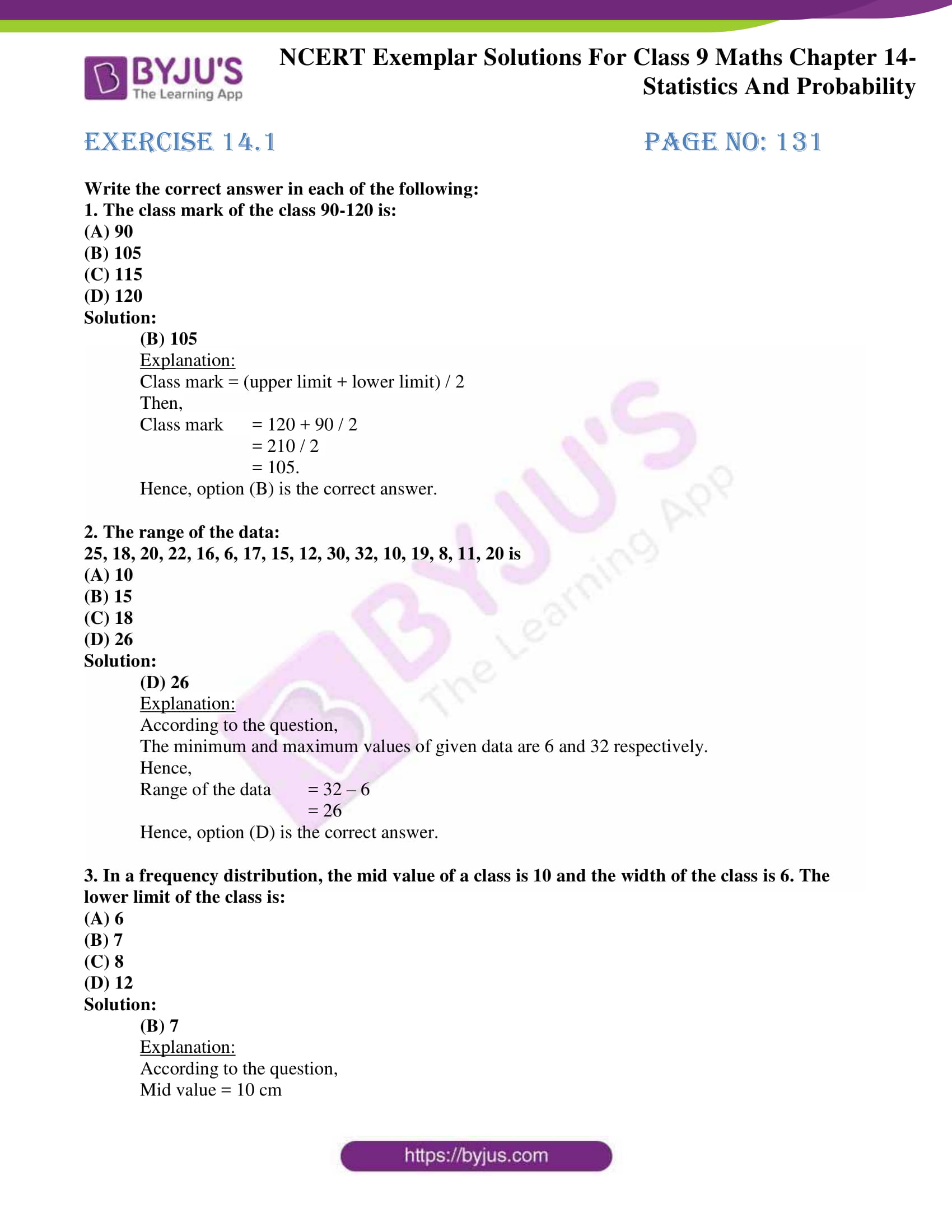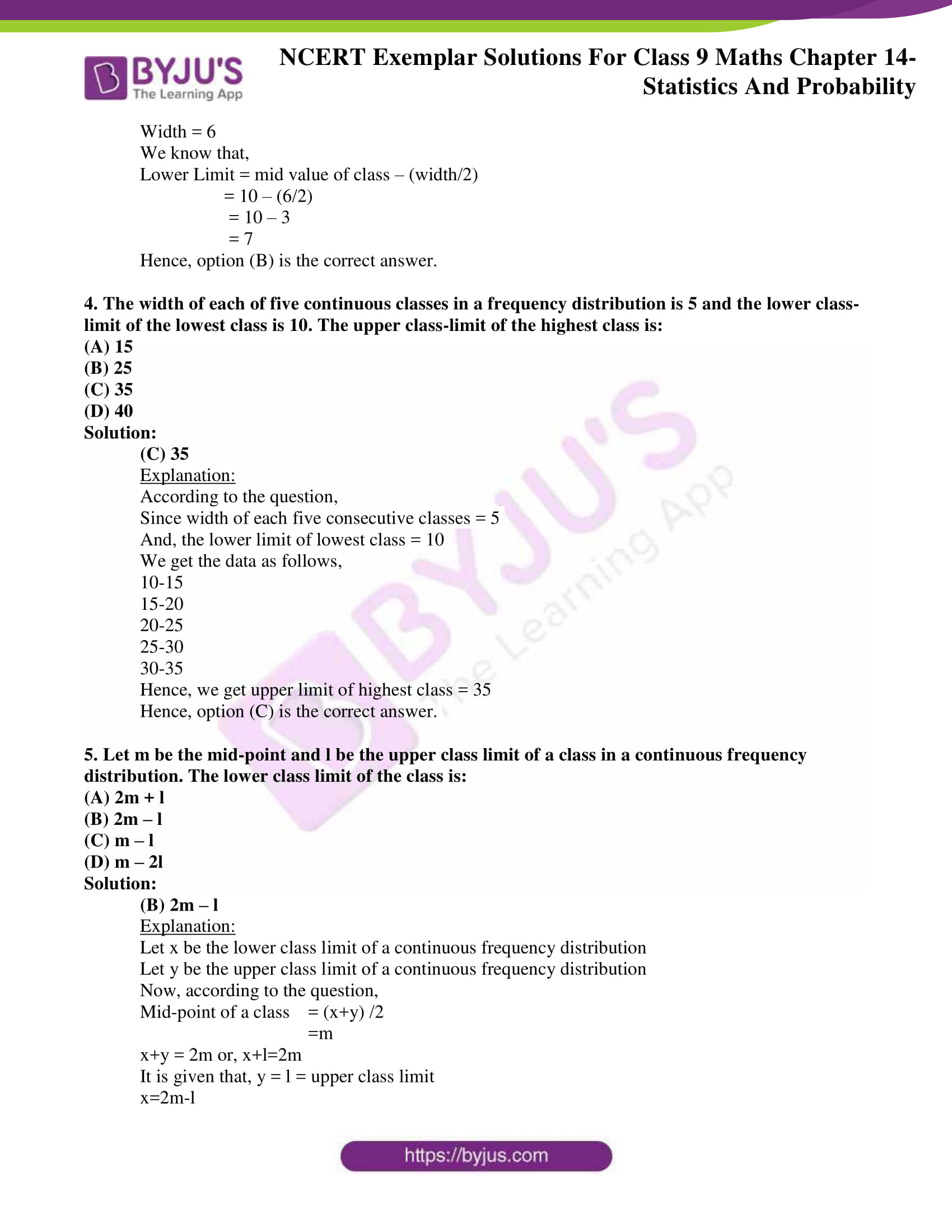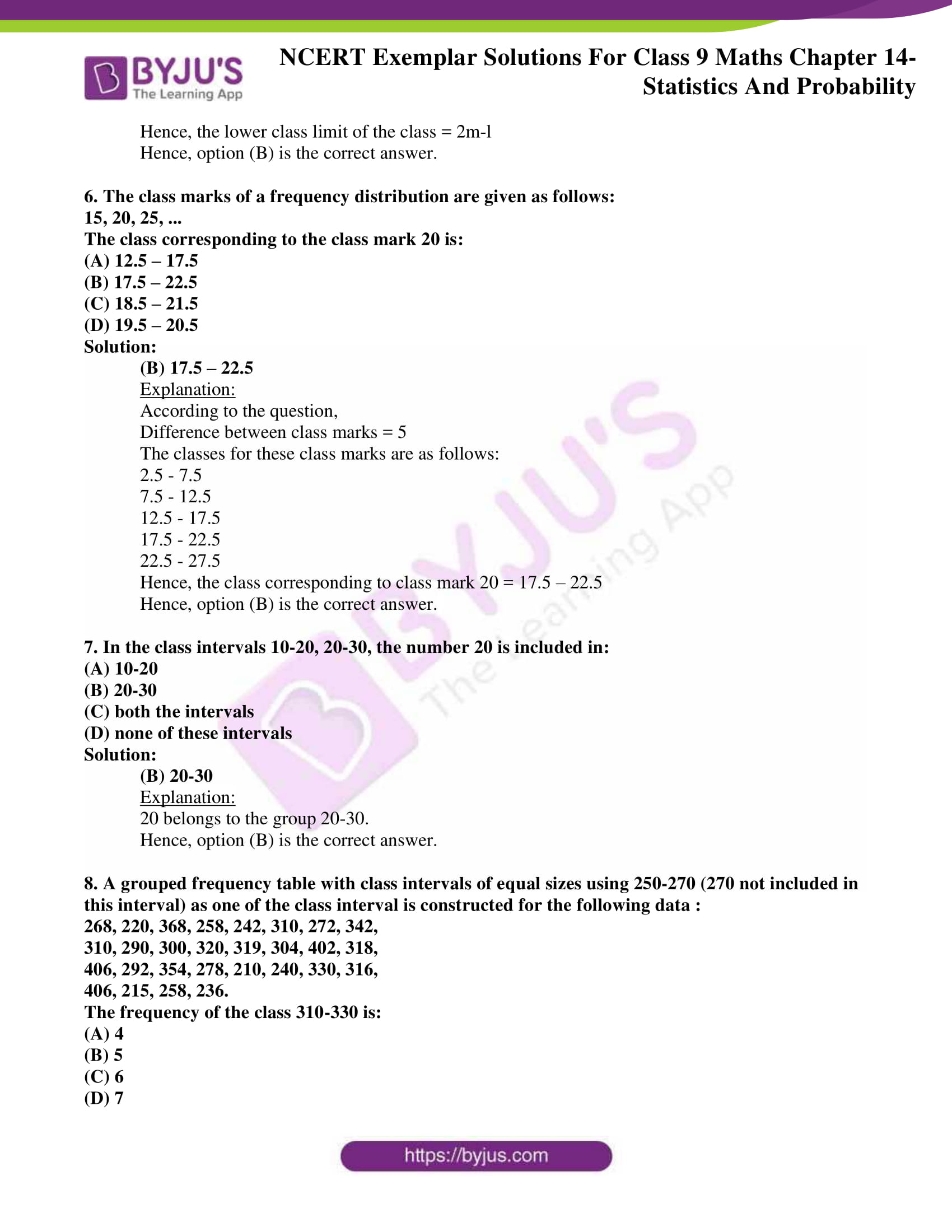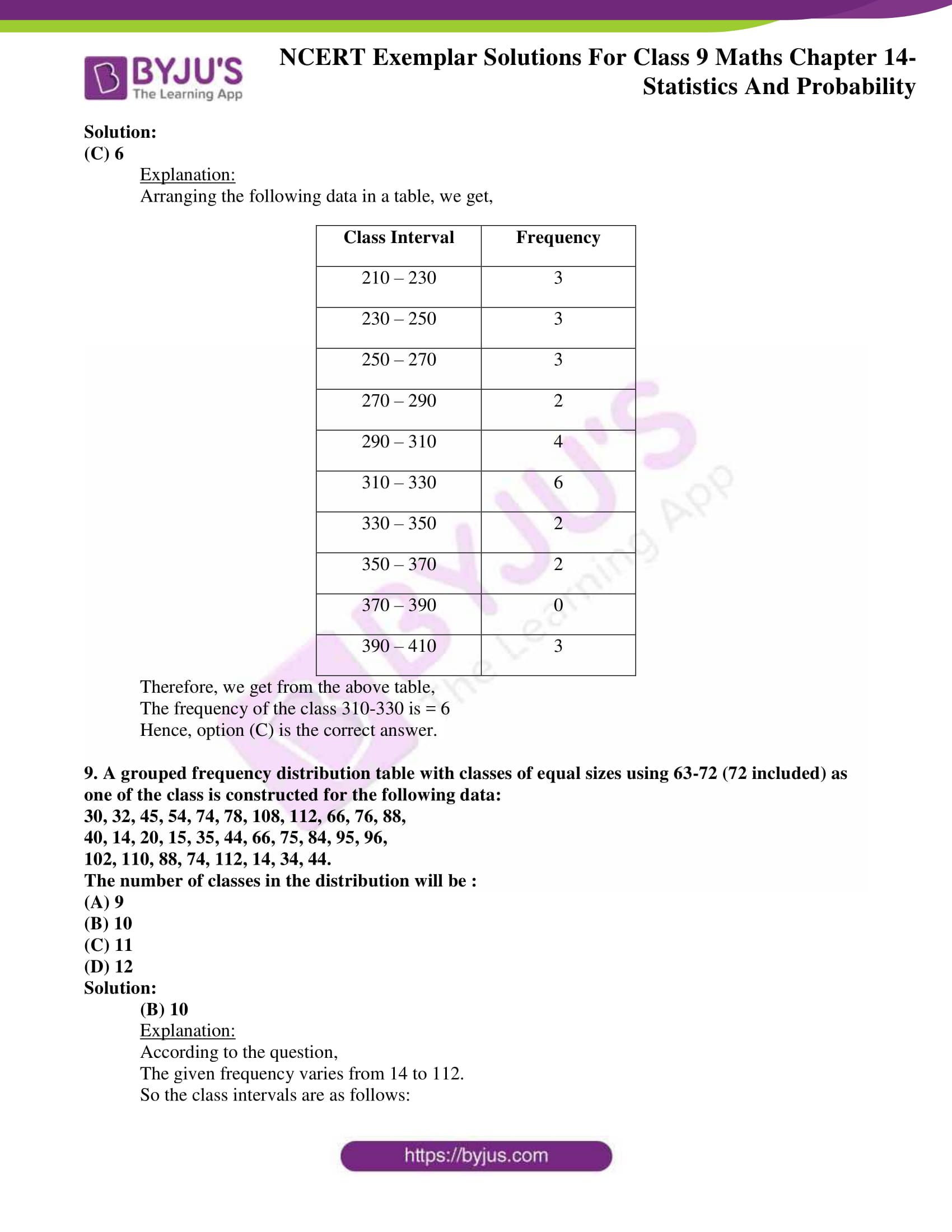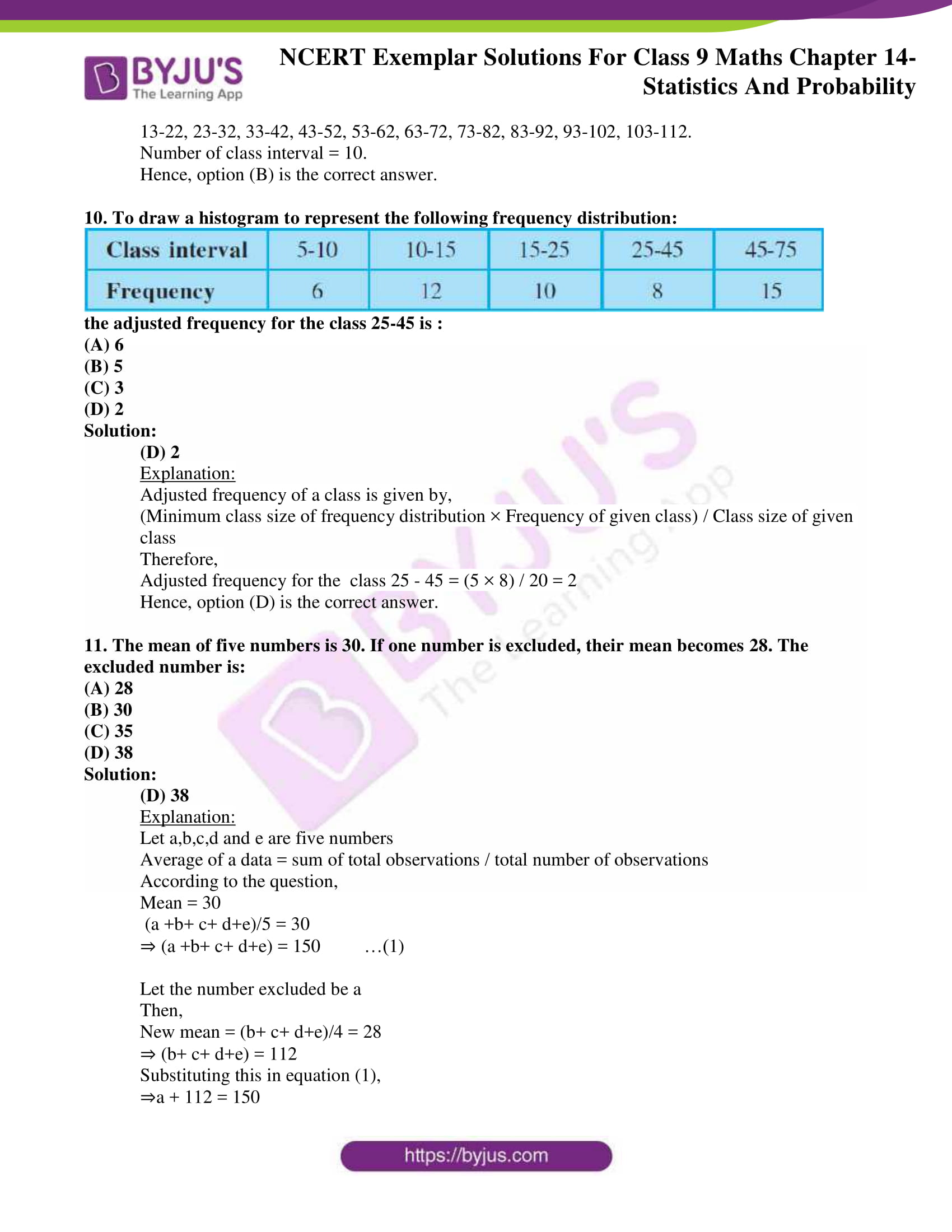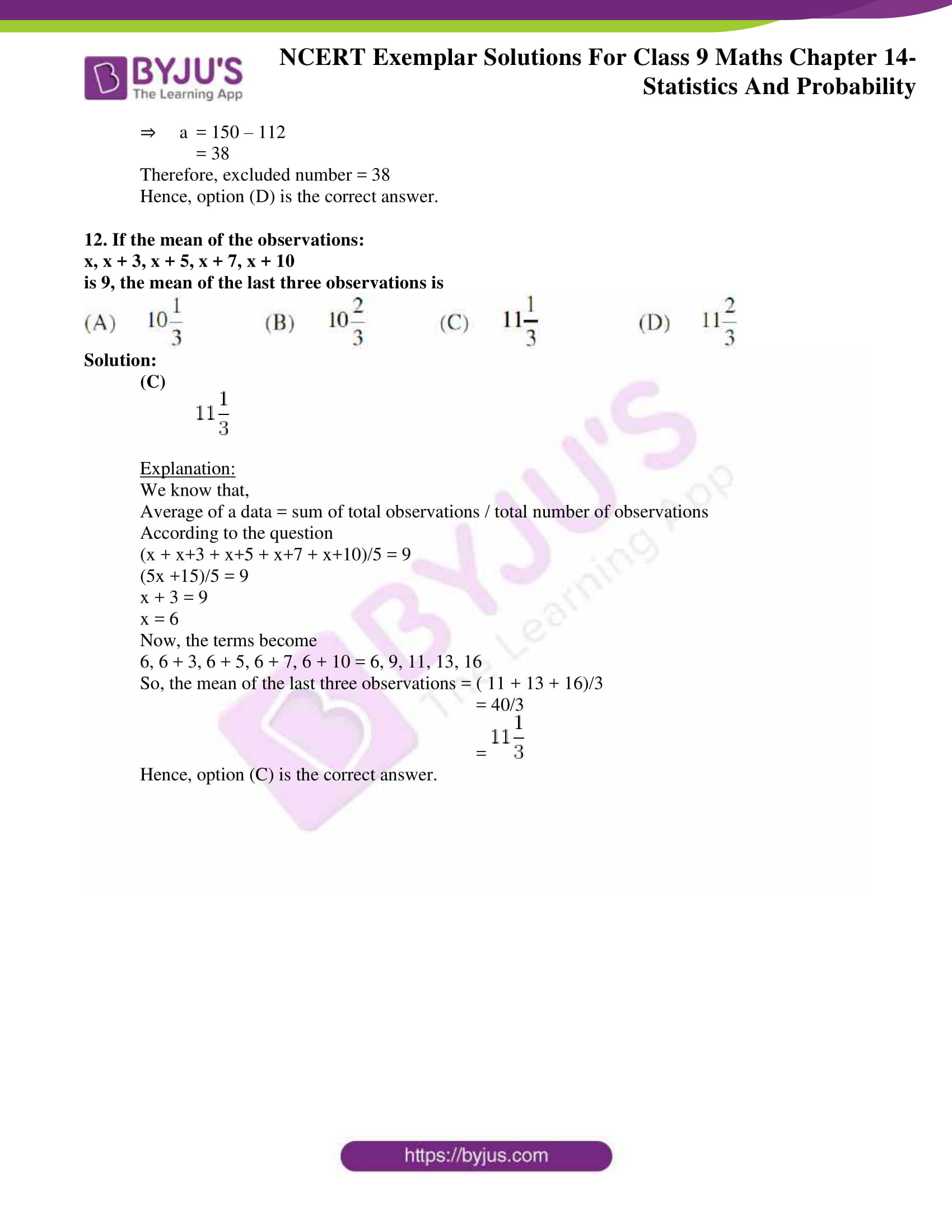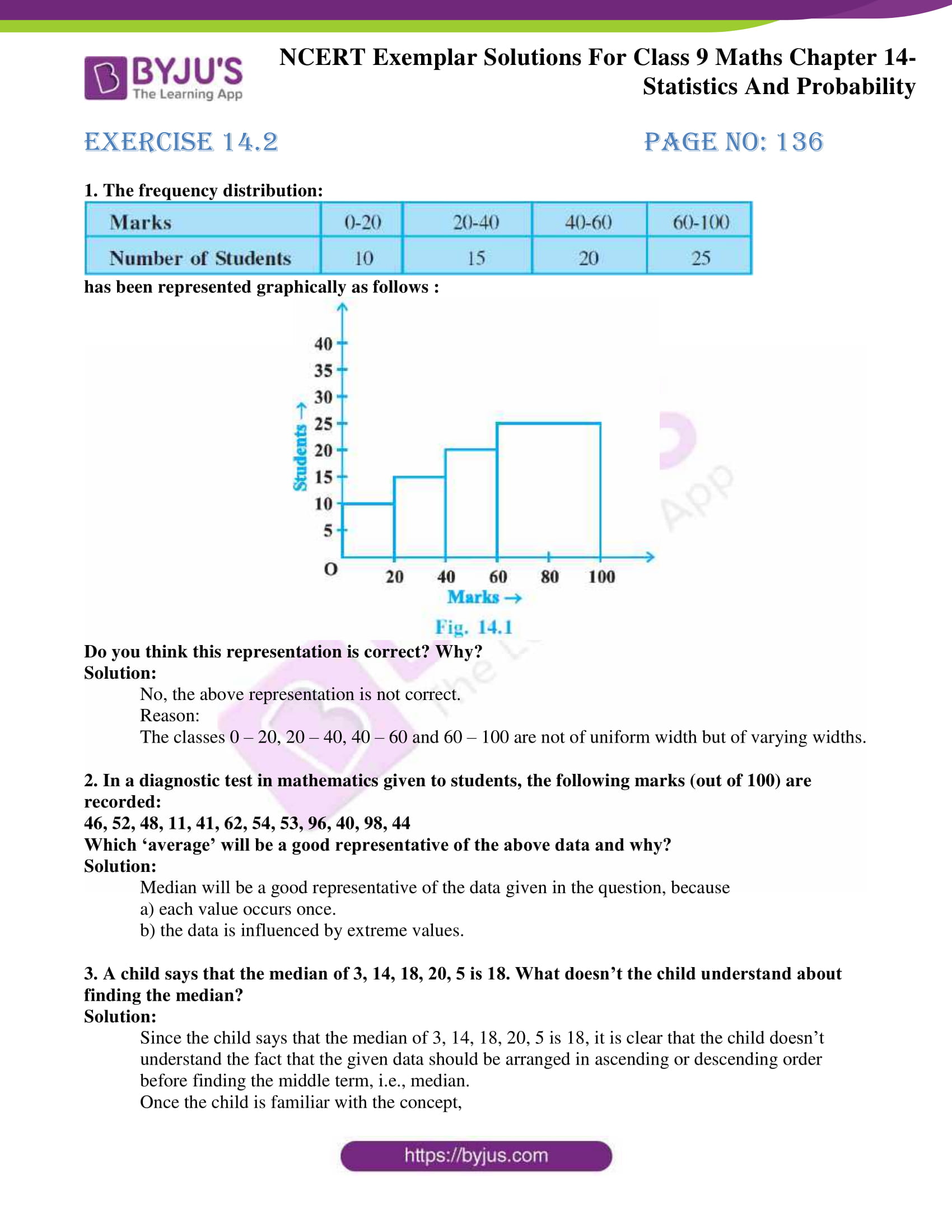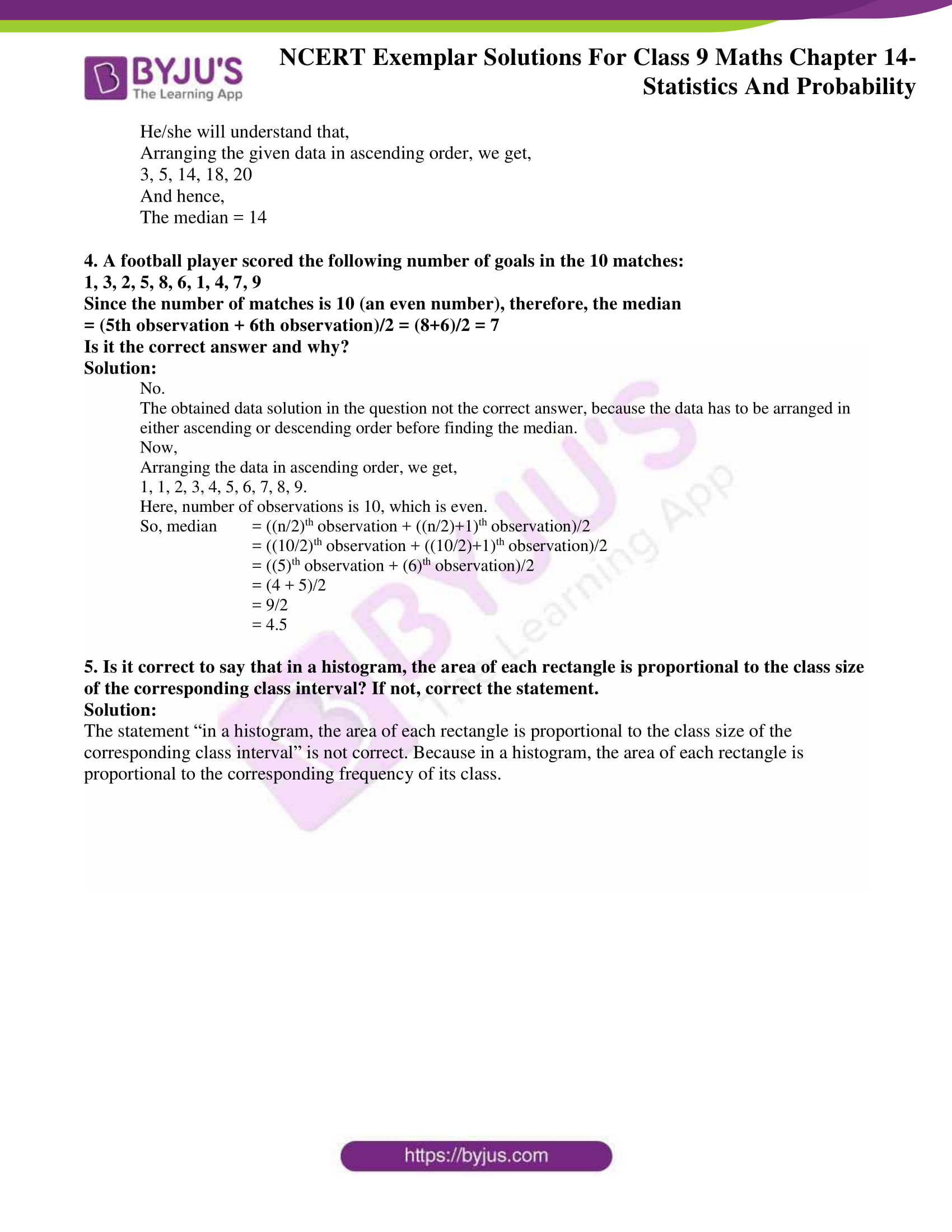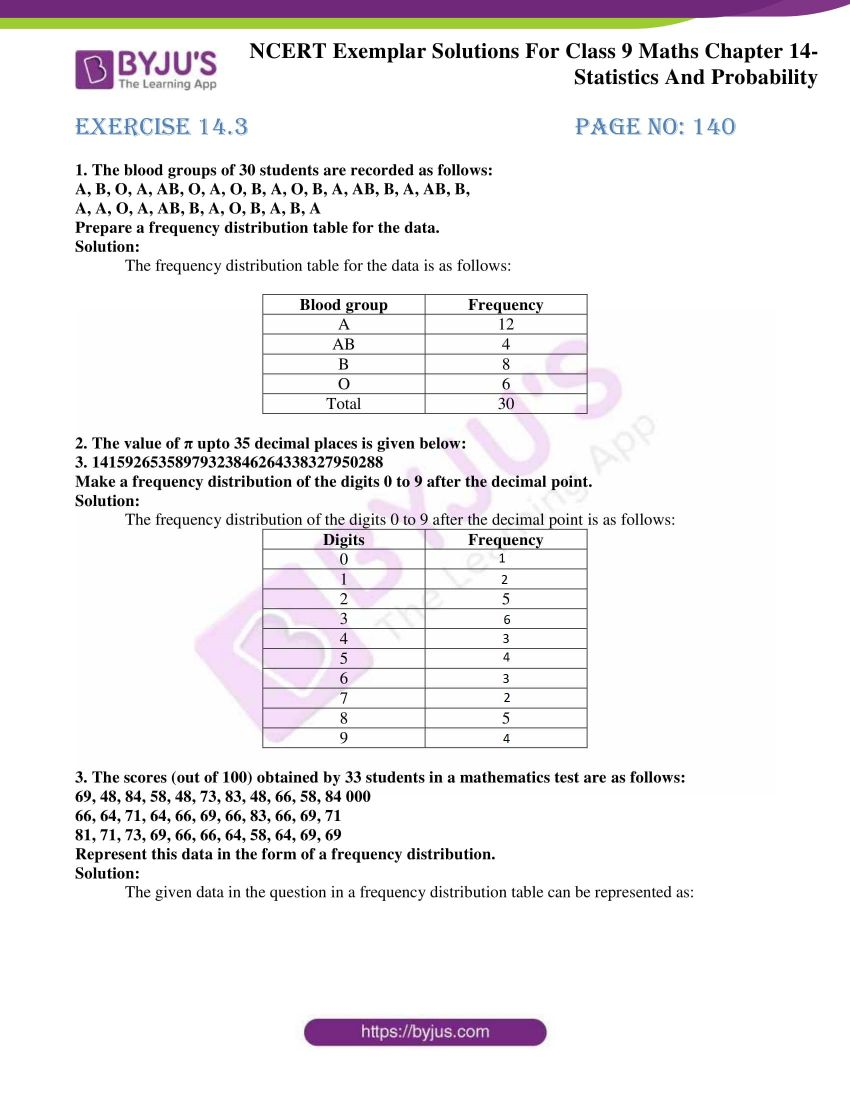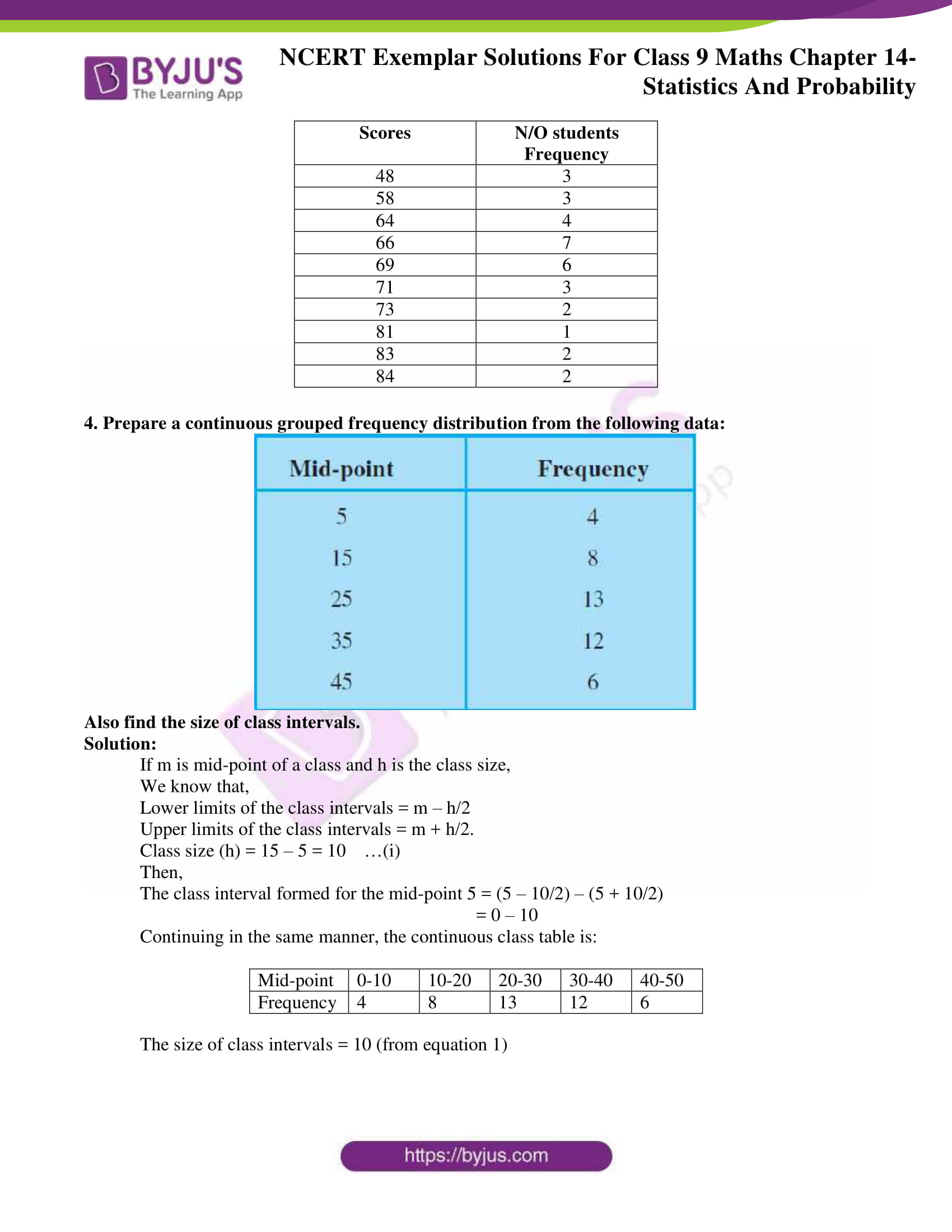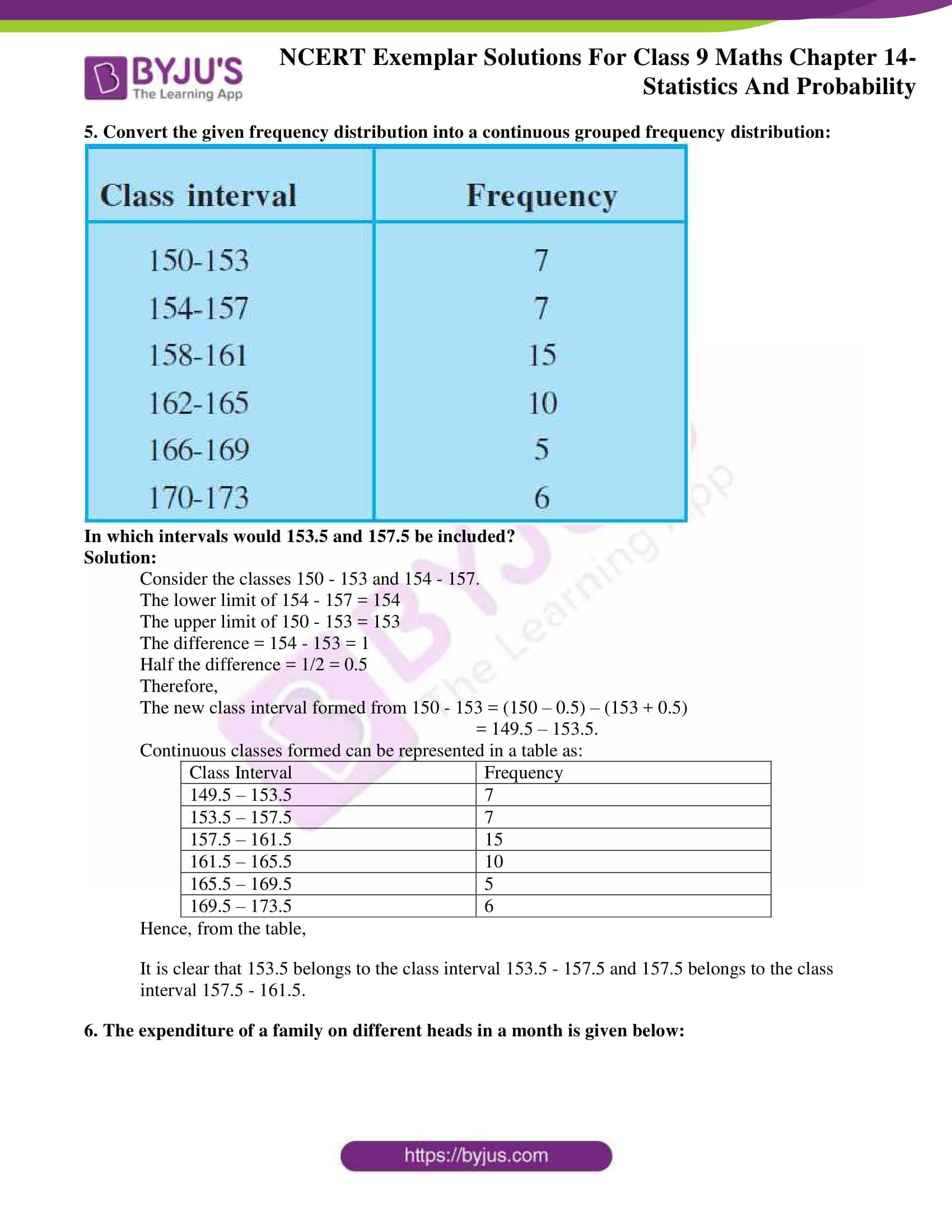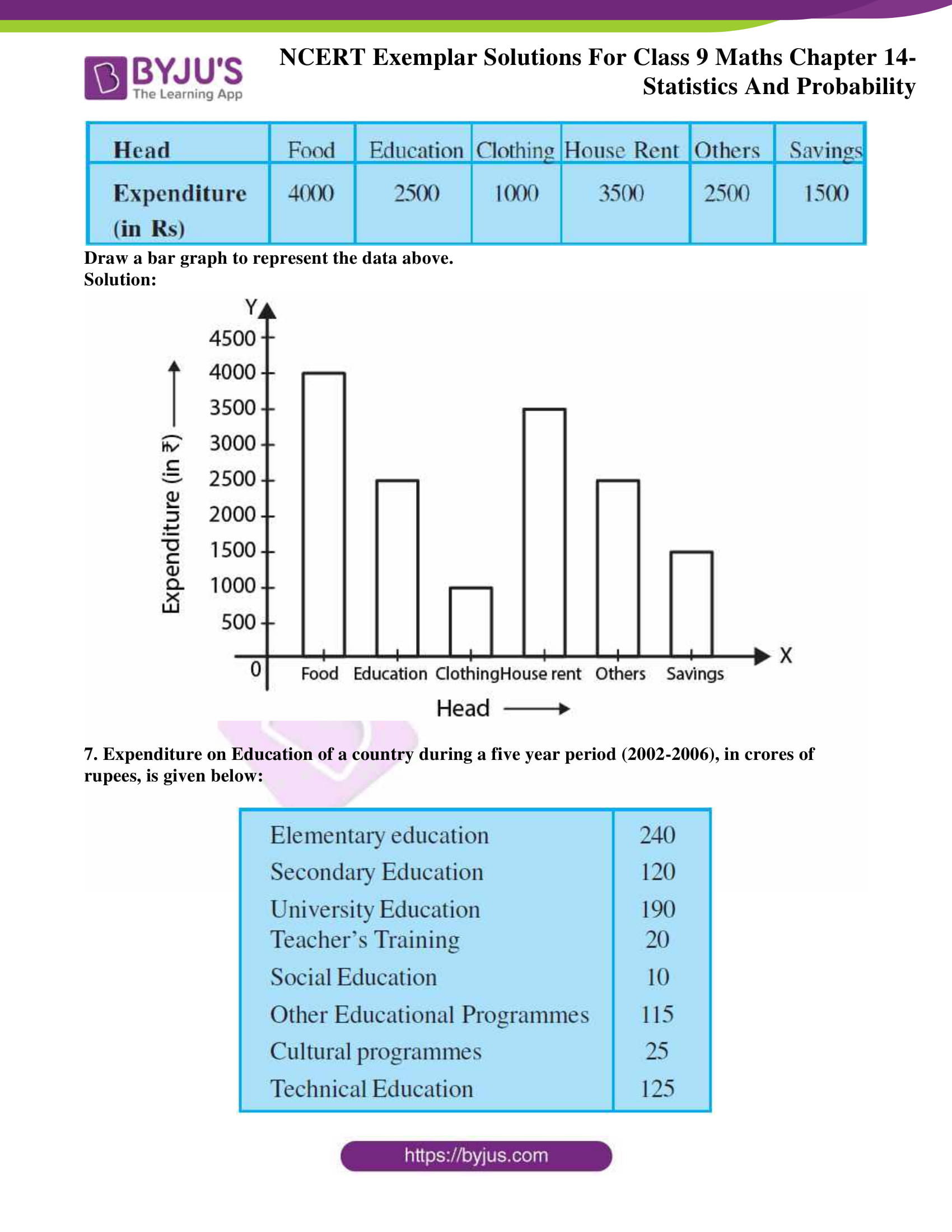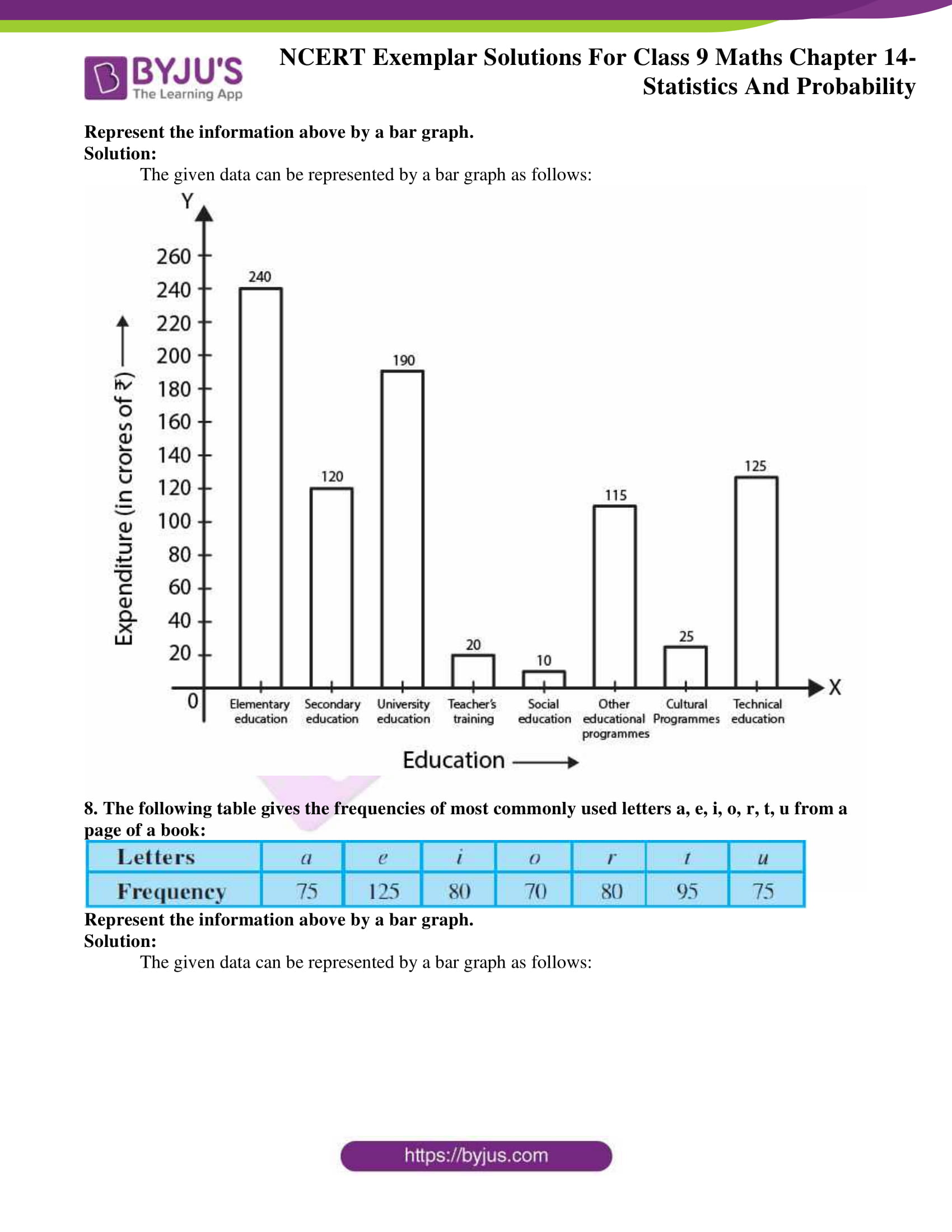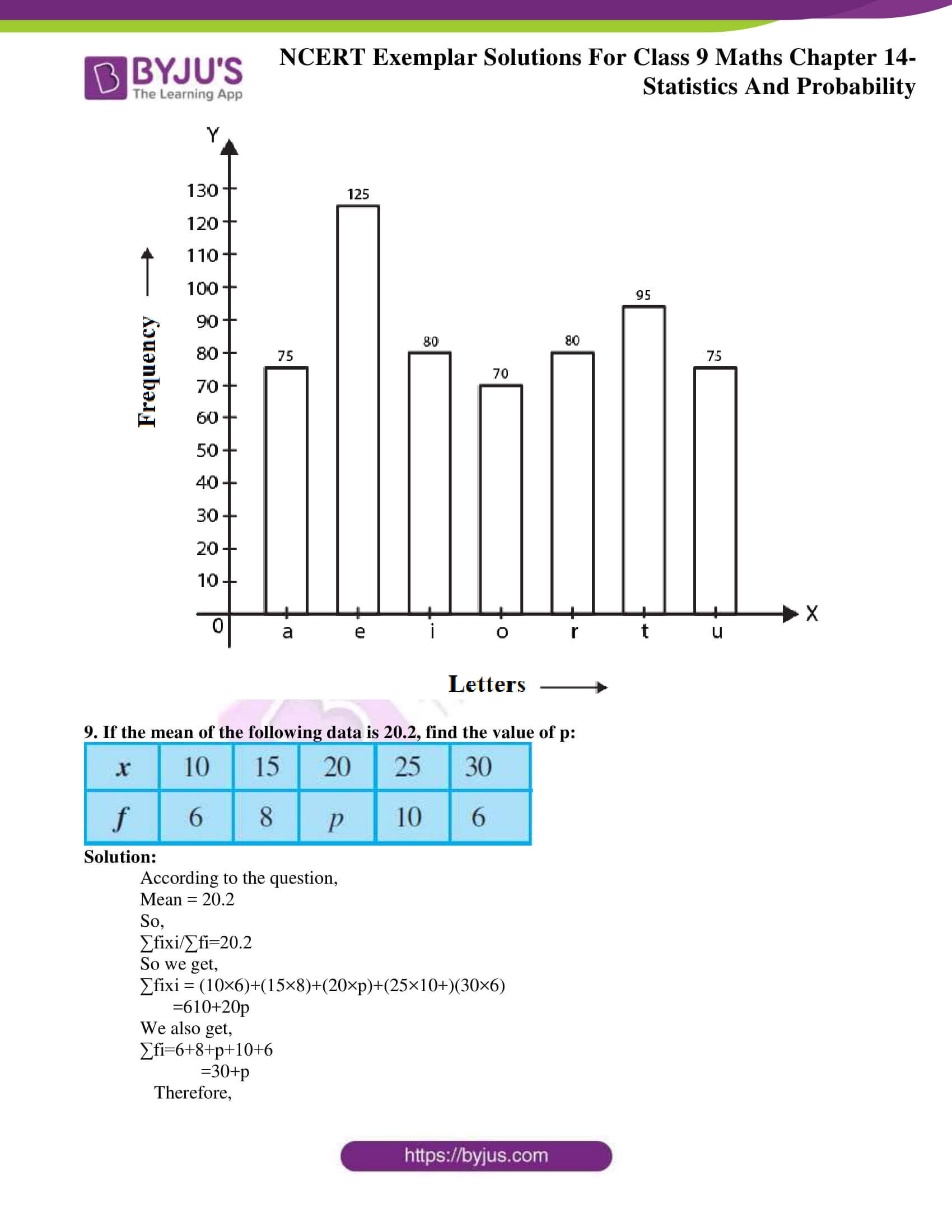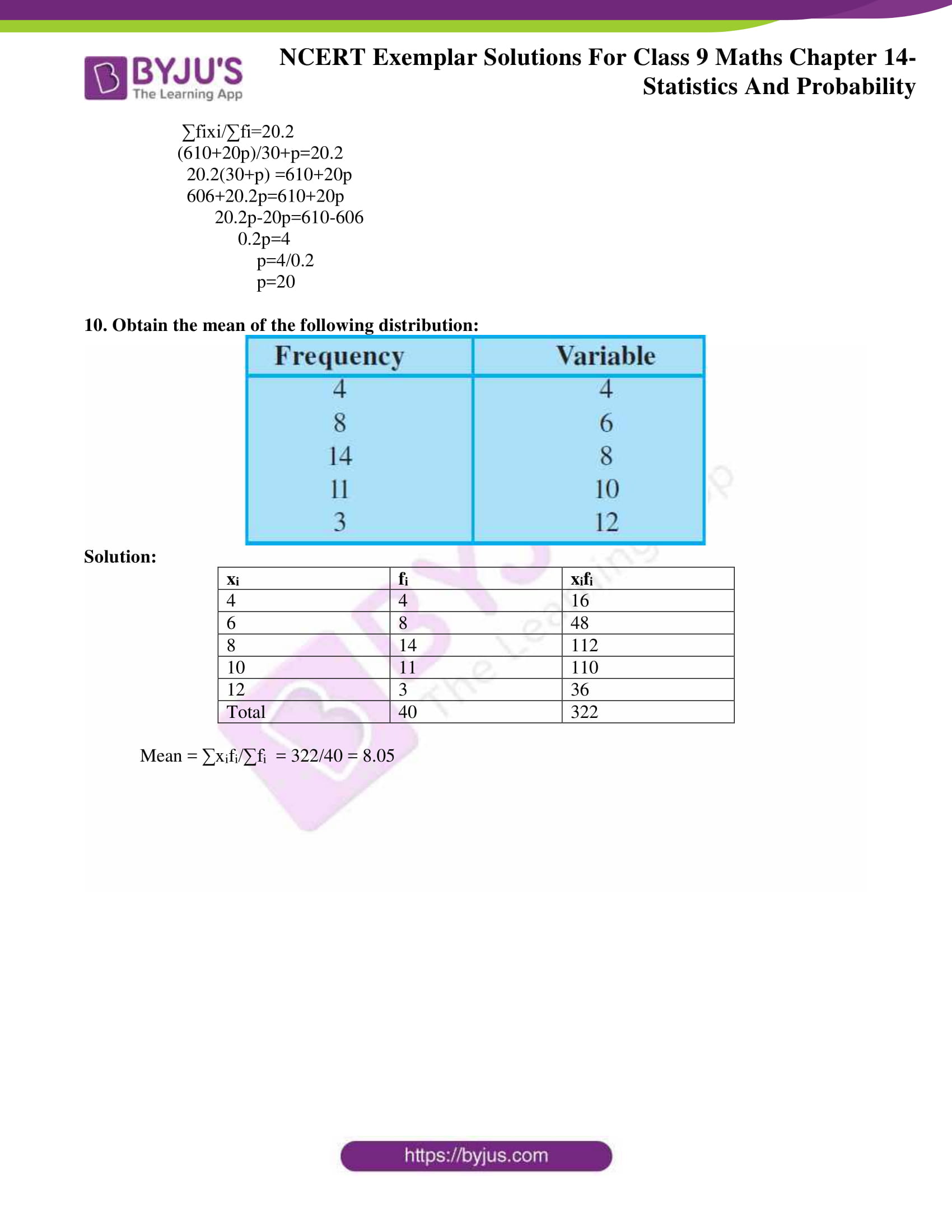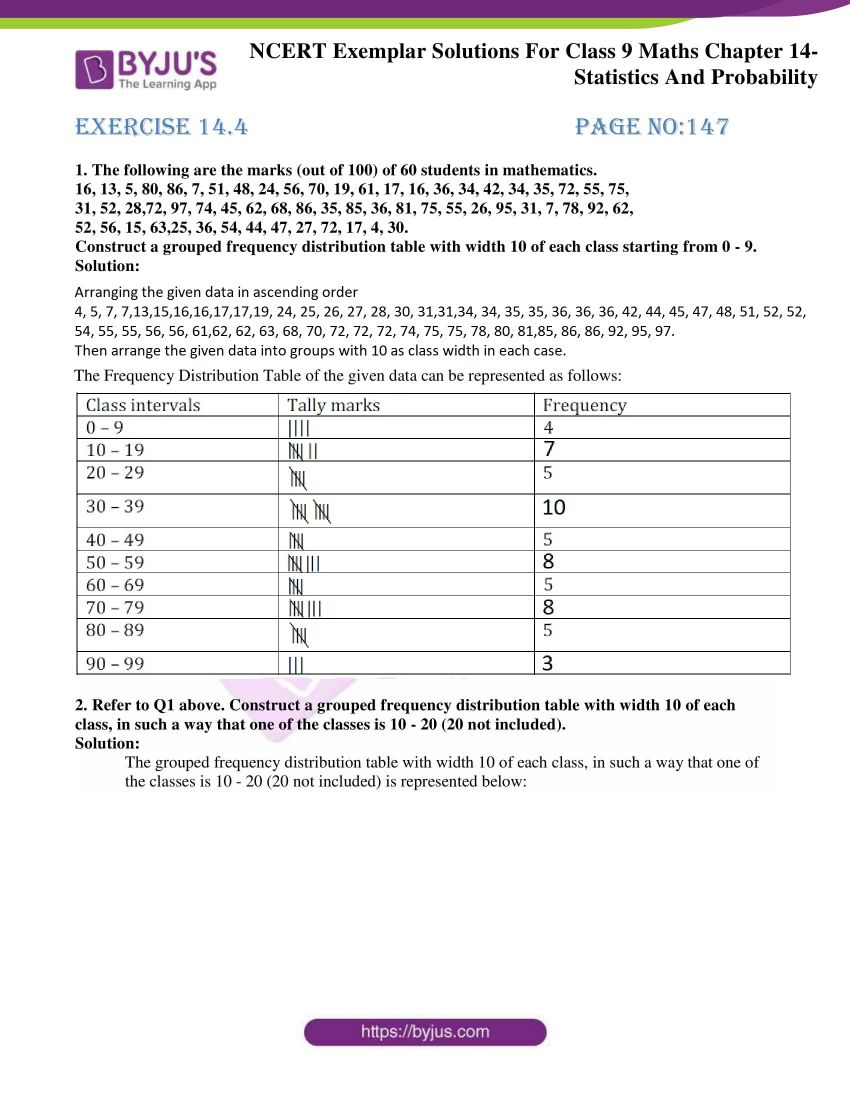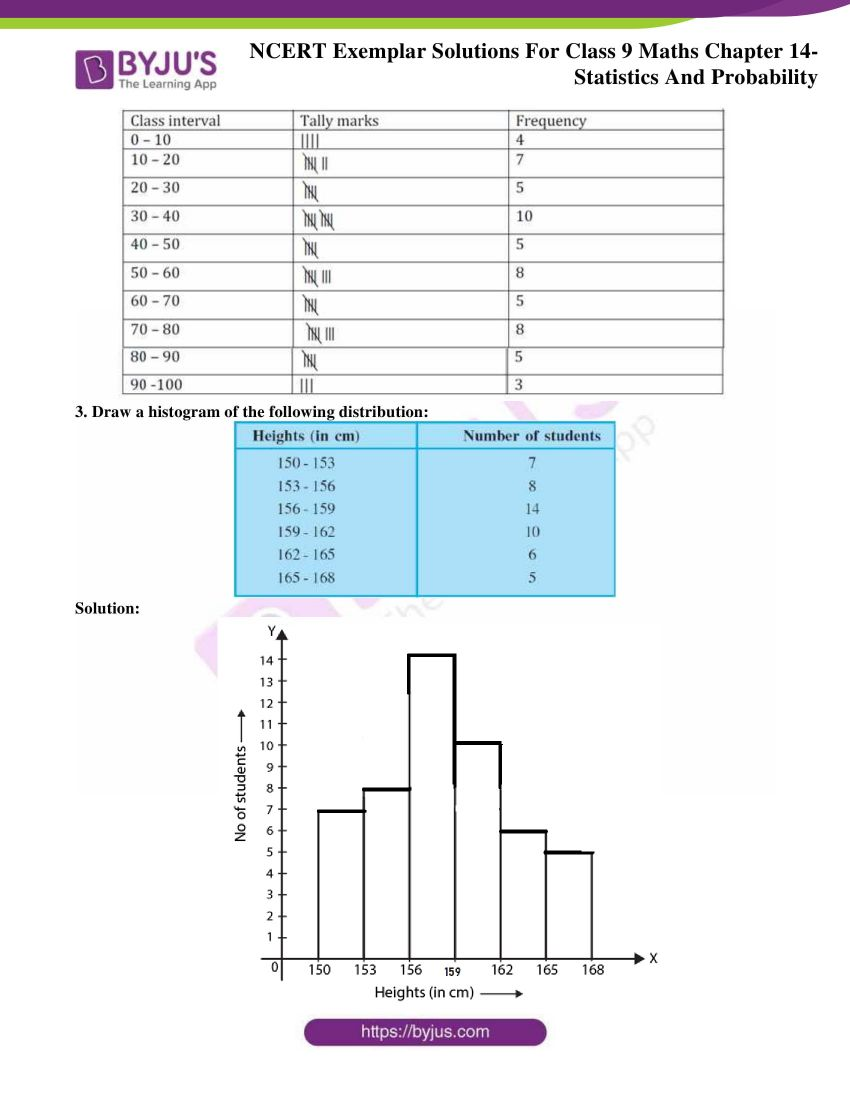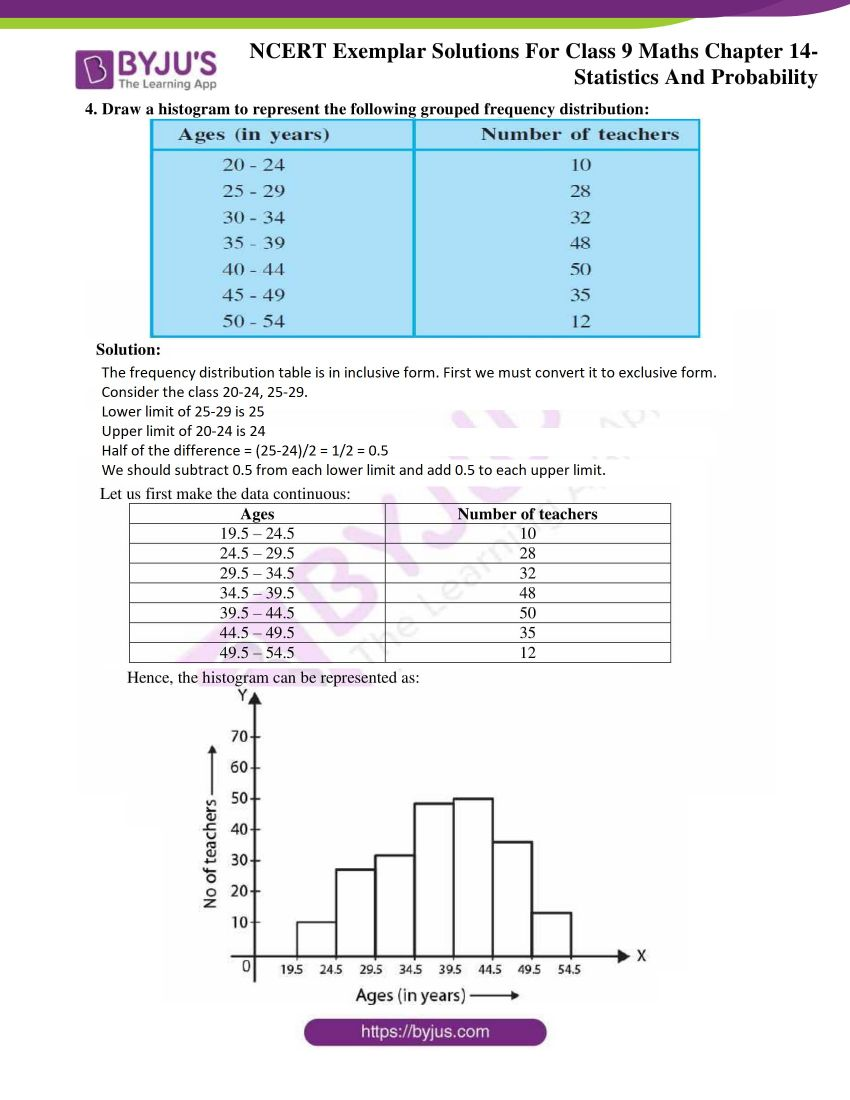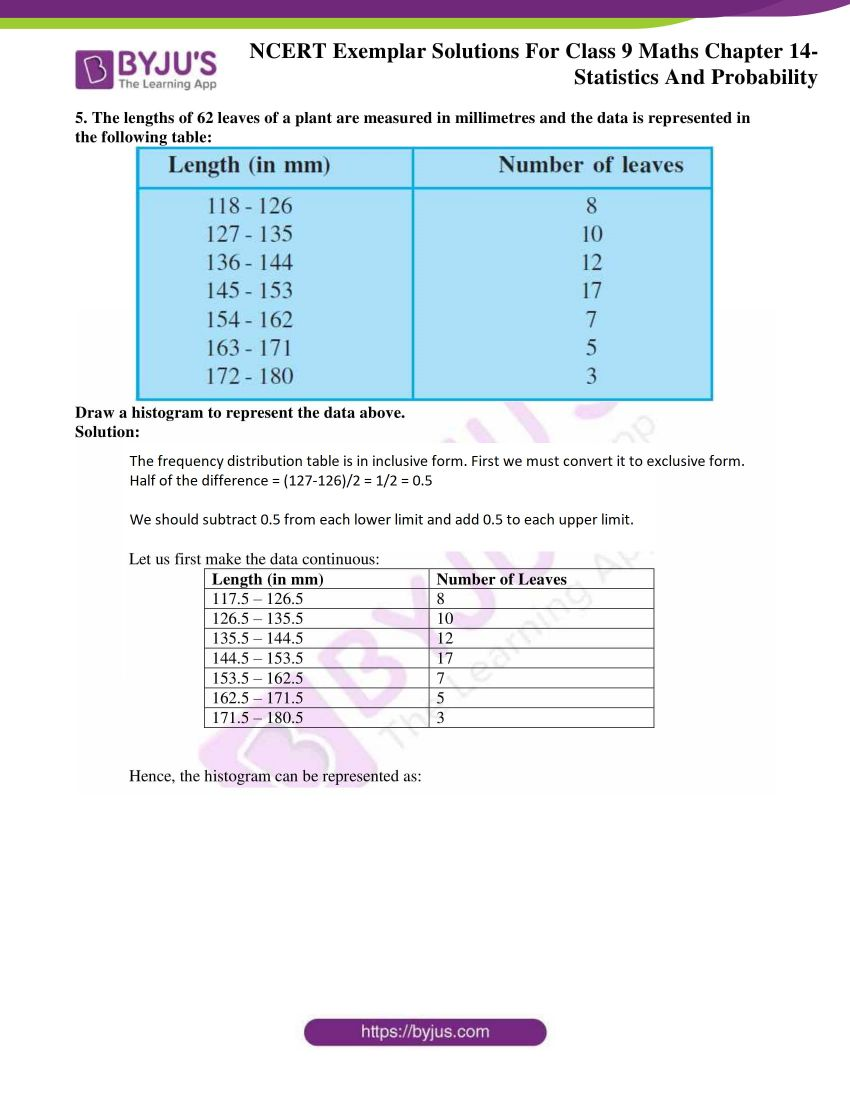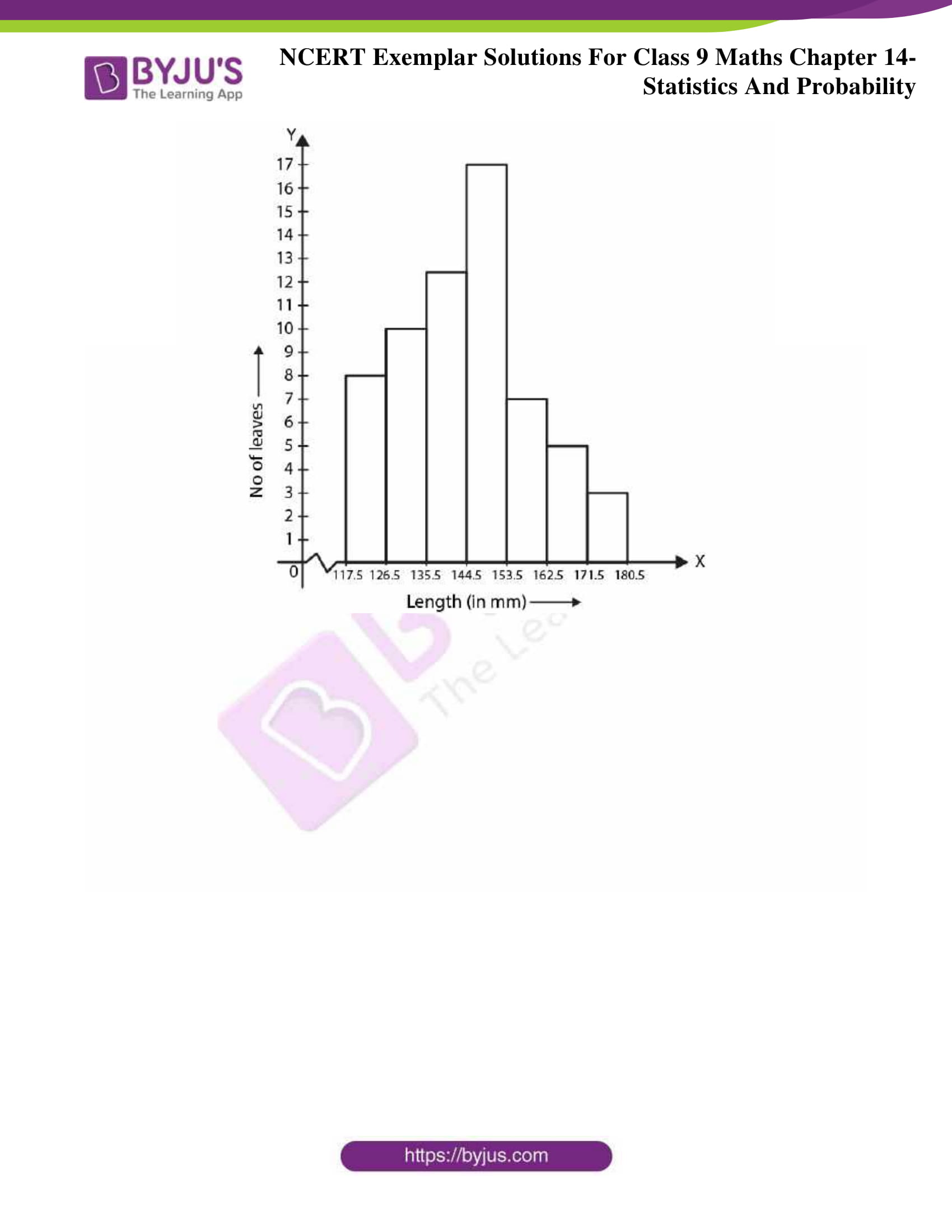### Access answers to NCERT Exemplar Solutions for Class 9 Maths Chapter 14 Statistics and Probability

Exercise 14.1 Page No: 131

Write the correct answer in each of the following:

1. The class mark of the class 90-120 is:

(A) 90

(B) 105

(C) 115

(D) 120

Solution:

(B) 105

Explanation:

Class mark = (upper limit + lower limit) / 2

Then,

Class mark = 120 + 90 / 2

= 210 / 2

= 105.

Hence, option (B) is the correct answer.

2. The range of the data:

25, 18, 20, 22, 16, 6, 17, 15, 12, 30, 32, 10, 19, 8, 11, 20 is

(A) 10

(B) 15

(C) 18

(D) 26

Solution:

(D) 26

Explanation:

According to the question,

The minimum and maximum values of given data are 6 and 32 respectively.

Hence,

Range of the data = 32 – 6

= 26

Hence, option (D) is the correct answer.

3. In a frequency distribution, the mid value of a class is 10 and the width of the class is 6. The lower limit of the class is:

(A) 6

(B) 7

(C) 8

(D) 12

Solution:

(B) 7

Explanation:

According to the question,

Mid value = 10 cm

Width = 6

We know that,

Lower Limit = mid value of class – (width/2)

= 10 – (6/2)

= 10 – 3

= 7

Hence, option (B) is the correct answer.

4. The width of each of five continuous classes in a frequency distribution is 5 and the lower class-limit of the lowest class is 10. The upper class-limit of the highest class is:

(A) 15

(B) 25

(C) 35

(D) 40

Solution:

(C) 35

Explanation:

According to the question,

Since width of each five consecutive classes = 5

And, the lower limit of lowest class = 10

We get the data as follows,

10-15

15-20

20-25

25-30

30-35

Hence, we get upper limit of highest class = 35

Hence, option (C) is the correct answer.

5. Let m be the mid-point and l be the upper class limit of a class in a continuous frequency distribution. The lower class limit of the class is:

(A) 2m + l

(B) 2m – l

(C) m – l

(D) m – 2l

Solution:

(B) 2m – l

Explanation:

Let x be the lower class limit of a continuous frequency distribution

Let y be the upper class limit of a continuous frequency distribution

Now, according to the question,

Mid-point of a class = (x+y) /2

=m

x+y = 2m or, x+l=2m

It is given that, y = l = upper class limit

x=2m-l

Hence, the lower class limit of the class = 2m-l

Hence, option (B) is the correct answer.

6. The class marks of a frequency distribution are given as follows:

15, 20, 25, …

The class corresponding to the class mark 20 is:

(A) 12.5 – 17.5

(B) 17.5 – 22.5

(C) 18.5 – 21.5

(D) 19.5 – 20.5

Solution:

(B) 17.5 – 22.5

Explanation:

According to the question,

Difference between class marks = 5

The classes for these class marks are as follows:

2.5 – 7.5

7.5 – 12.5

12.5 – 17.5

17.5 – 22.5

22.5 – 27.5

Hence, the class corresponding to class mark 20 = 17.5 – 22.5

Hence, option (B) is the correct answer.

7. In the class intervals 10-20, 20-30, the number 20 is included in:

(A) 10-20

(B) 20-30

(C) both the intervals

(D) none of these intervals

Solution:

(B) 20-30

Explanation:

20 belongs to the group 20-30.

Hence, option (B) is the correct answer.

8. A grouped frequency table with class intervals of equal sizes using 250-270 (270 not included in this interval) as one of the class interval is constructed for the following data :

268, 220, 368, 258, 242, 310, 272, 342,

310, 290, 300, 320, 319, 304, 402, 318,

406, 292, 354, 278, 210, 240, 330, 316,

406, 215, 258, 236.

The frequency of the class 310-330 is:

(A) 4

(B) 5

(C) 6

(D) 7

Solution:

(C) 6

Explanation:

Arranging the following data in a table, we get,

 Class Interval Frequency 210 – 230 3 230 – 250 3 250 – 270 3 270 – 290 2 290 – 310 4 310 – 330 6 330 – 350 2 350 – 370 2 370 – 390 0 390 – 410 3

Therefore, we get from the above table,

The frequency of the class 310-330 is = 6

Hence, option (C) is the correct answer.

9. A grouped frequency distribution table with classes of equal sizes using 63-72 (72 included) as one of the class is constructed for the following data:

30, 32, 45, 54, 74, 78, 108, 112, 66, 76, 88,

40, 14, 20, 15, 35, 44, 66, 75, 84, 95, 96,

102, 110, 88, 74, 112, 14, 34, 44.

The number of classes in the distribution will be :

(A) 9

(B) 10

(C) 11

(D) 12

Solution:

(B) 10

Explanation:

According to the question,

The given frequency varies from 14 to 112.

So the class intervals are as follows:

13-22, 23-32, 33-42, 43-52, 53-62, 63-72, 73-82, 83-92, 93-102, 103-112.

Number of class interval = 10.

Hence, option (B) is the correct answer.

10. To draw a histogram to represent the following frequency distribution: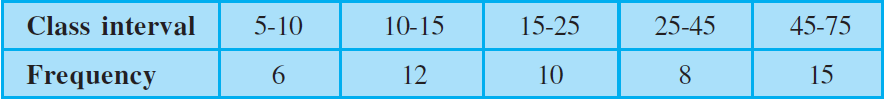the adjusted frequency for the class 25-45 is :

(A) 6

(B) 5

(C) 3

(D) 2

Solution:

(D) 2

Explanation:

Adjusted frequency of a class is given by,

(Minimum class size of frequency distribution × Frequency of given class) / Class size of given class

Therefore,

Adjusted frequency for the  class 25 – 45 = (5 × 8) / 20 = 2

Hence, option (D) is the correct answer.

11. The mean of five numbers is 30. If one number is excluded, their mean becomes 28. The excluded number is:

(A) 28

(B) 30

(C) 35

(D) 38

Solution:

(D) 38

Explanation:

Let a,b,c,d and e are five numbers

Average of a data = sum of total observations / total number of observations

According to the question,

Mean = 30

(a +b+ c+ d+e)/5 = 30

⇒ (a +b+ c+ d+e) = 150 …(1)

Let the number excluded be a

Then,

New mean = (b+ c+ d+e)/4 = 28

⇒ (b+ c+ d+e) = 112

Substituting this in equation (1),

⇒a + 112 = 150

⇒ a = 150 – 112

= 38

Therefore, excluded number = 38

Hence, option (D) is the correct answer.

12. If the mean of the observations:

x, x + 3, x + 5, x + 7, x + 10

is 9, the mean of the last three observations is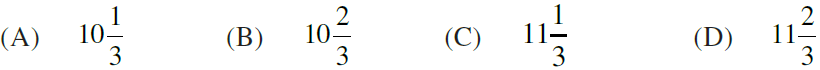Solution:

(C)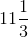Explanation:

We know that,

Average of a data = sum of total observations / total number of observations

According to the question

(x + x+3 + x+5 + x+7 + x+10)/5 = 9

(5x +15)/5 = 9

x + 3 = 9

x = 6

Now, the terms become

6, 6 + 3, 6 + 5, 6 + 7, 6 + 10 = 6, 9, 11, 13, 16

So, the mean of the last three observations = ( 11 + 13 + 16)/3

= 40/3

=Hence, option (C) is the correct answer.

Exercise 14.2 Page No: 136

1. The frequency distribution: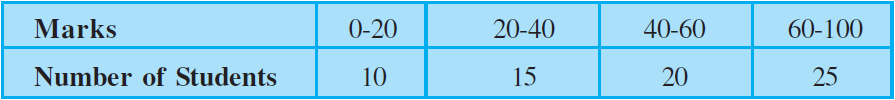has been represented graphically as follows :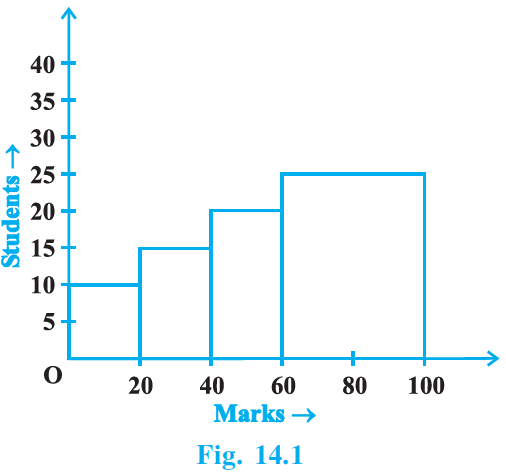Do you think this representation is correct? Why?

Solution:

No, the above representation is not correct.

Reason:

The classes 0 – 20, 20 – 40, 40 – 60 and 60 – 100 are not of uniform width but of varying widths.

2. In a diagnostic test in mathematics given to students, the following marks (out of 100) are recorded:

46, 52, 48, 11, 41, 62, 54, 53, 96, 40, 98, 44

Which ‘average’ will be a good representative of the above data and why?

Solution:

Median will be a good representative of the data given in the question, because

a) each value occurs once.

b) the data is influenced by extreme values.

3. A child says that the median of 3, 14, 18, 20, 5 is 18. What doesn’t the child understand about finding the median?

Solution:

Since the child says that the median of 3, 14, 18, 20, 5 is 18, it is clear that the child doesn’t understand the fact that the given data should be arranged in ascending or descending order before finding the middle term, i.e., median.

Once the child is familiar with the concept,

He/she will understand that,

Arranging the given data in ascending order, we get,

3, 5, 14, 18, 20

And hence,

The median = 14

4. A football player scored the following number of goals in the 10 matches:

1, 3, 2, 5, 8, 6, 1, 4, 7, 9

Since the number of matches is 10 (an even number), therefore, the median

= (5th observation + 6th observation)/2 = (8+6)/2 = 7

Is it the correct answer and why?

Solution:

No.

The obtained data solution in the question not the correct answer, because the data has to be arranged in either ascending or descending order before finding the median.

Now,

Arranging the data in ascending order, we get,

1, 1, 2, 3, 4, 5, 6, 7, 8, 9.

Here, number of observations is 10, which is even.

So, median = ((n/2)th observation + ((n/2)+1)th observation)/2

= ((10/2)th observation + ((10/2)+1)th observation)/2

= ((5)th observation + (6)th observation)/2

= (4 + 5)/2

= 9/2

= 4.5

5. Is it correct to say that in a histogram, the area of each rectangle is proportional to the class size of the corresponding class interval? If not, correct the statement.

Solution:

The statement “in a histogram, the area of each rectangle is proportional to the class size of the corresponding class interval” is not correct. Because in a histogram, the area of each rectangle is proportional to the corresponding frequency of its class.

Exercise 14.3 Page No: 140

1. The blood groups of 30 students are recorded as follows:

A, B, O, A, AB, O, A, O, B, A, O, B, A, AB, B, A, AB, B,

A, A, O, A, AB, B, A, O, B, A, B, A

Prepare a frequency distribution table for the data.

Solution:

The frequency distribution table for the data is as follows:

 Blood group Frequency A 12 AB 4 B 8 O 6 Total 30

2. The value of π upto 35 decimal places is given below:

3. 14159265358979323846264338327950288

Make a frequency distribution of the digits 0 to 9 after the decimal point.

Solution:

The frequency distribution of the digits 0 to 9 after the decimal point is as follows:

 Digits Frequency 0 1 1 2 2 5 3 6 4 3 5 4 6 3 7 2 8 5 9 4

3. The scores (out of 100) obtained by 33 students in a mathematics test are as follows:

69, 48, 84, 58, 48, 73, 83, 48, 66, 58, 84 000

66, 64, 71, 64, 66, 69, 66, 83, 66, 69, 71

81, 71, 73, 69, 66, 66, 64, 58, 64, 69, 69

Represent this data in the form of a frequency distribution.

Solution:

The given data in the question in a frequency distribution table can be represented as:

 Scores N/O students Frequency 48 3 58 3 64 4 66 7 69 6 71 3 73 2 81 1 83 2 84 2

4. Prepare a continuous grouped frequency distribution from the following data: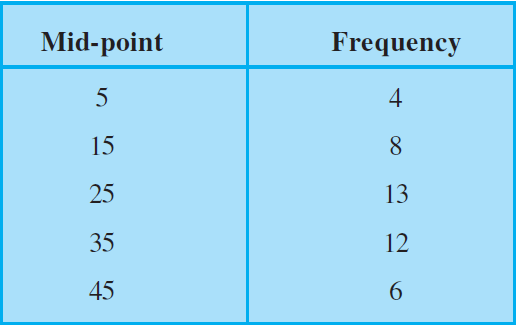Also find the size of class intervals.

Solution:

If m is mid-point of a class and h is the class size,

We know that,

Lower limits of the class intervals = m – h/2

Upper limits of the class intervals = m + h/2.

Class size (h) = 15 – 5 = 10 …(i)

Then,

The class interval formed for the mid-point 5 = (5 – 10/2) – (5 + 10/2)

= 0 – 10

Continuing in the same manner, the continuous class table is:

 Mid-point 0-10 10-20 20-30 30-40 40-50 Frequency 4 8 13 12 6

The size of class intervals = 10 (from equation 1)

5. Convert the given frequency distribution into a continuous grouped frequency distribution: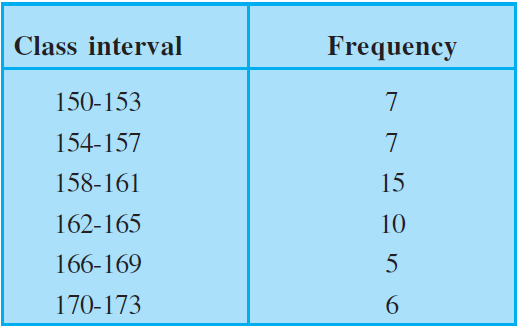In which intervals would 153.5 and 157.5 be included?

Solution:

Consider the classes 150 – 153 and 154 – 157.

The lower limit of 154 – 157 = 154

The upper limit of 150 – 153 = 153

The difference = 154 – 153 = 1

Half the difference = 1/2 = 0.5

Therefore,

The new class interval formed from 150 – 153 = (150 – 0.5) – (153 + 0.5)

= 149.5 – 153.5.

Continuous classes formed can be represented in a table as:

 Class Interval Frequency 149.5 – 153.5 7 153.5 – 157.5 7 157.5 – 161.5 15 161.5 – 165.5 10 165.5 – 169.5 5 169.5 – 173.5 6

Hence, from the table,

It is clear that 153.5 belongs to the class interval 153.5 – 157.5 and 157.5 belongs to the class interval 157.5 – 161.5.

6. The expenditure of a family on different heads in a month is given below: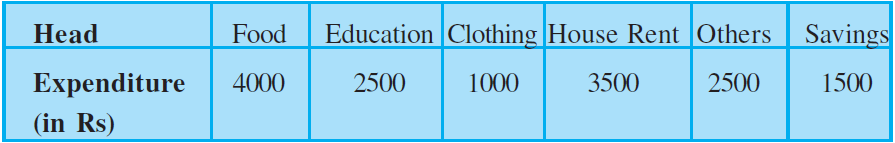Draw a bar graph to represent the data above.

Solution: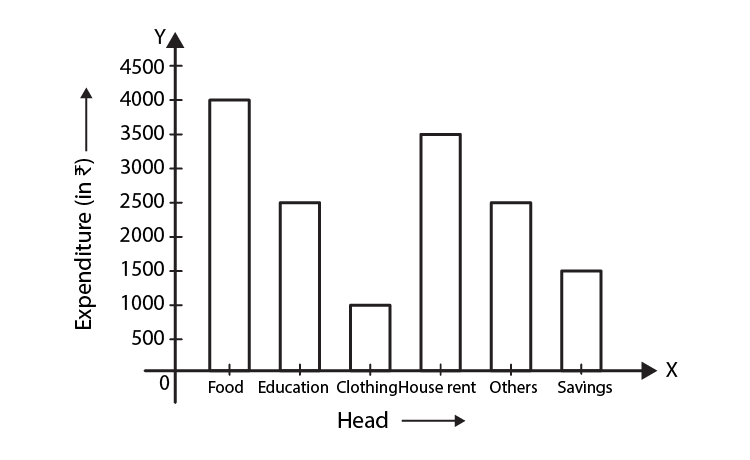7. Expenditure on Education of a country during a five year period (2002-2006), in crores of rupees, is given below: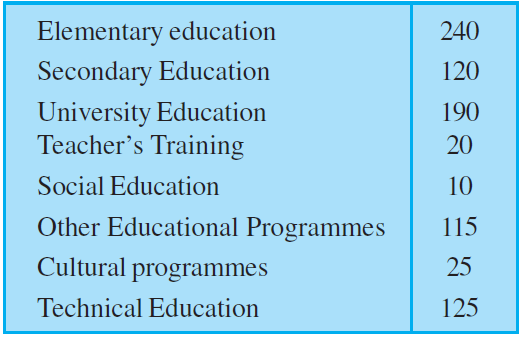Represent the information above by a bar graph.

Solution:

The given data can be represented by a bar graph as follows: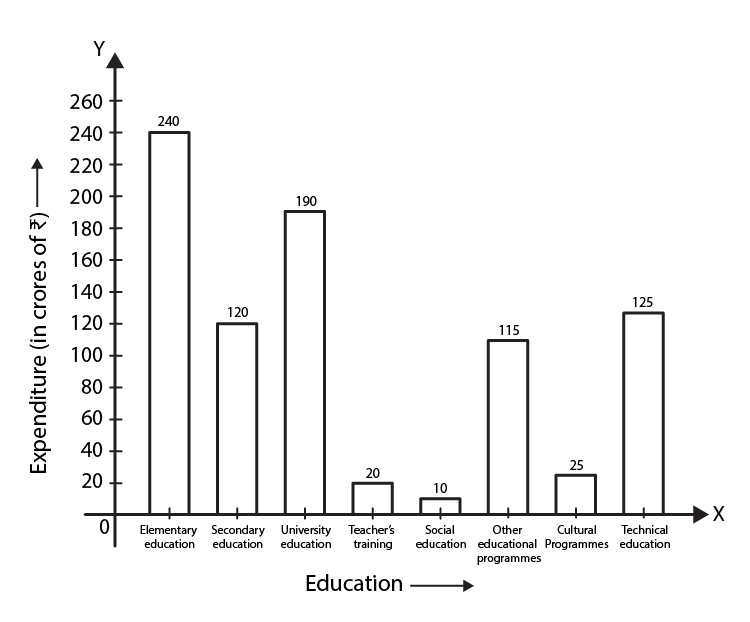8. The following table gives the frequencies of most commonly used letters a, e, i, o, r, t, u from a page of a book: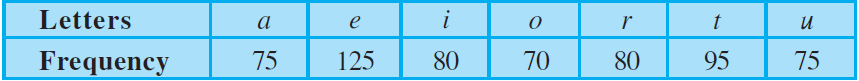Represent the information above by a bar graph.

Solution:

The given data can be represented by a bar graph as follows: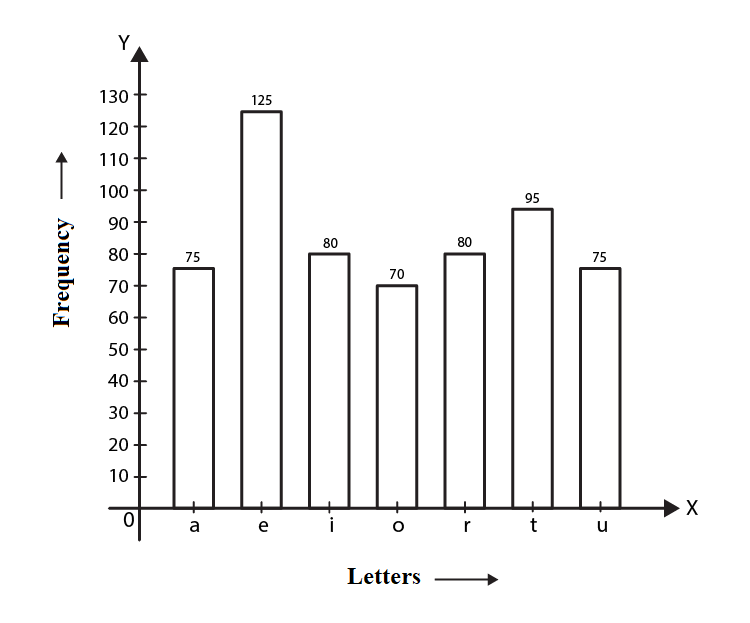9. If the mean of the following data is 20.2, find the value of p: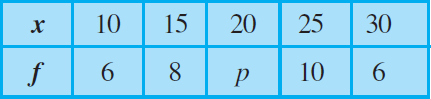Solution:

According to the question,

Mean = 20.2

So,

∑fixi/∑fi=20.2

So we get,

∑fixi = (10×6)+(15×8)+(20×p)+(25×10+)(30×6)

=610+20p

We also get,

∑fi=6+8+p+10+6

=30+p

Therefore,

∑fixi/∑fi=20.2

(610+20p)/30+p=20.2

20.2(30+p) =610+20p

606+20.2p=610+20p

20.2p-20p=610-606

0.2p=4

p=4/0.2

p=20

10. Obtain the mean of the following distribution: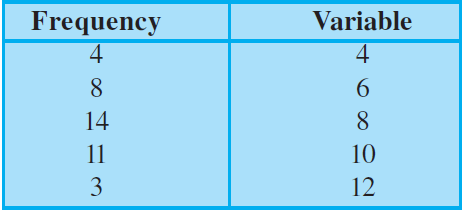Solution:

 xi fi xifi 4 4 16 6 8 48 8 14 112 10 11 110 12 3 36 Total 40 322

Mean = ∑xifi/∑fi = 322/40 = 8.05

Exercise 14.4 Page No:147

1. The following are the marks (out of 100) of 60 students in mathematics.

16, 13, 5, 80, 86, 7, 51, 48, 24, 56, 70, 19, 61, 17, 16, 36, 34, 42, 34, 35, 72, 55, 75,

31, 52, 28,72, 97, 74, 45, 62, 68, 86, 35, 85, 36, 81, 75, 55, 26, 95, 31, 7, 78, 92, 62,

52, 56, 15, 63,25, 36, 54, 44, 47, 27, 72, 17, 4, 30.

Construct a grouped frequency distribution table with width 10 of each class starting from 0 – 9.

Solution:

Arranging the given data in ascending order

4, 5, 7, 7,13,15,16,16,17,17,19, 24, 25, 26, 27, 28, 30, 31,31,34, 34, 35, 35, 36, 36, 36, 42, 44, 45, 47, 48, 51, 52, 52, 54, 55, 55, 56, 56, 61,62, 62, 63, 68, 70, 72, 72, 72, 74, 75, 75, 78, 80, 81,85, 86, 86, 92, 95, 97.

Then arrange the given data into groups with 10 as class width in each case.

The Frequency Distribution Table of the given data can be represented as follows: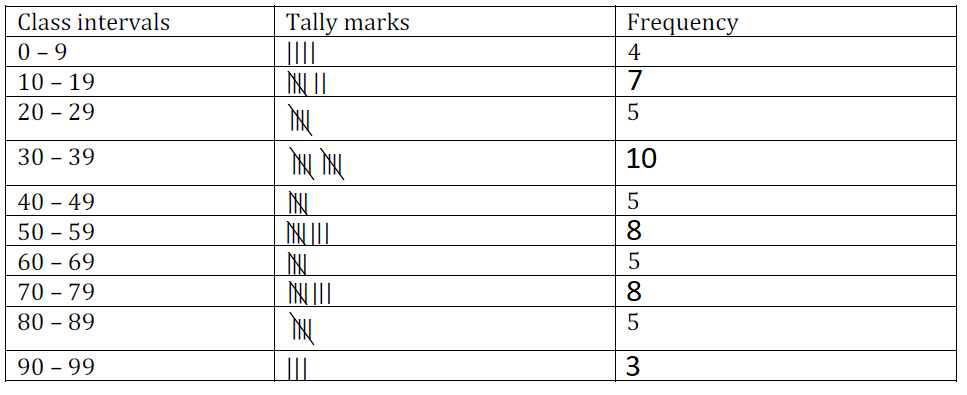2. Refer to Q1 above. Construct a grouped frequency distribution table with width 10 of each class, in such a way that one of the classes is 10 – 20 (20 not included).

Solution:

The grouped frequency distribution table with width 10 of each class, in such a way that one of the classes is 10 – 20 (20 not included) is represented below: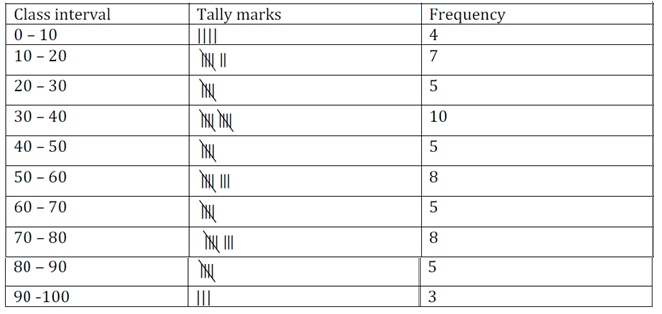3. Draw a histogram of the following distribution: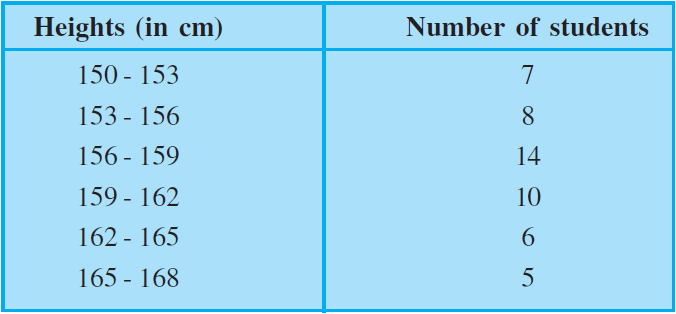Solution: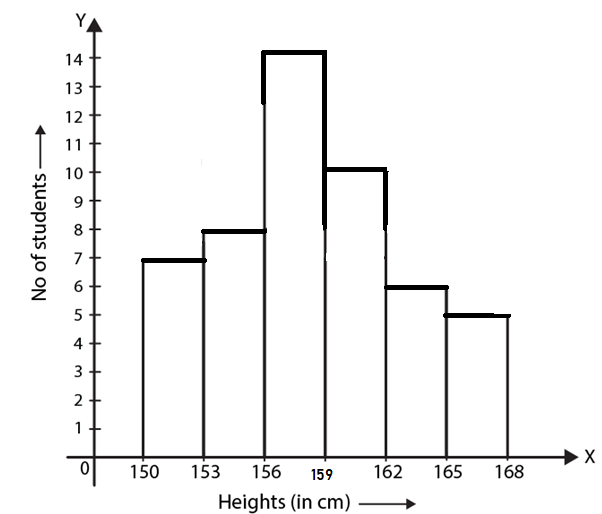4. Draw a histogram to represent the following grouped frequency distribution: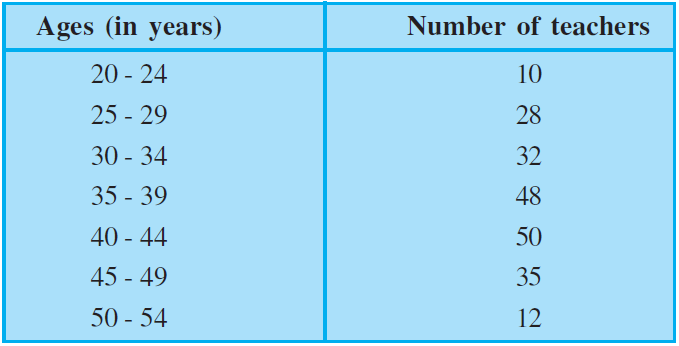Solution:

The frequency distribution table is in inclusive form. First we must convert it to exclusive form.

Consider the class 20-24, 25-29.

Lower limit of 25-29 is 25

Upper limit of 20-24 is 24

Half of the difference = (25-24)/2 = 1/2 = 0.5

We should subtract 0.5 from each lower limit and add 0.5 to each upper limit.

Let us first make the data continuous:

 Ages Number of teachers 19.5 – 24.5 10 24.5 – 29.5 28 29.5 – 34.5 32 34.5 – 39.5 48 39.5 – 44.5 50 44.5 – 49.5 35 49.5 – 54.5 12

Hence, the histogram can be represented as: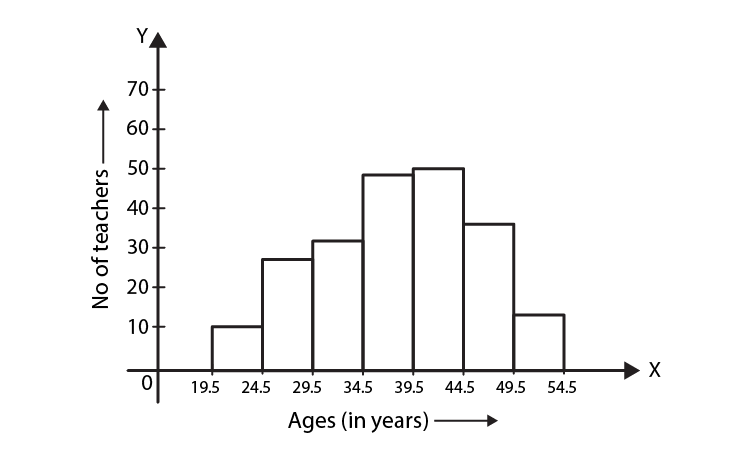5. The lengths of 62 leaves of a plant are measured in millimetres and the data is represented in the following table: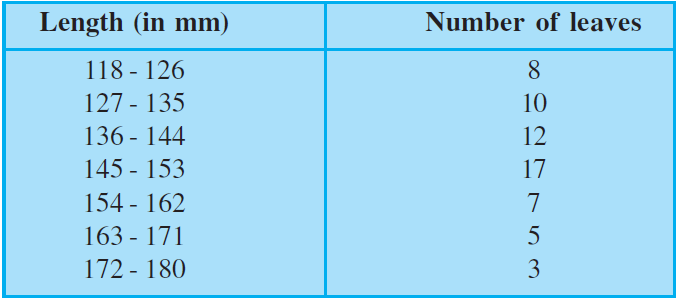Draw a histogram to represent the data above.

Solution:

The frequency distribution table is in inclusive form. First we must convert it to exclusive form.

Half of the difference = (127-126)/2 = 1/2 = 0.5

We should subtract 0.5 from each lower limit and add 0.5 to each upper limit.

Let us first make the data continuous:

 Length (in mm) Number of Leaves 117.5 – 126.5 8 126.5 – 135.5 10 135.5 – 144.5 12 144.5 – 153.5 17 153.5 – 162.5 7 162.5 – 171.5 5 171.5 – 180.5 3

Hence, the histogram can be represented as: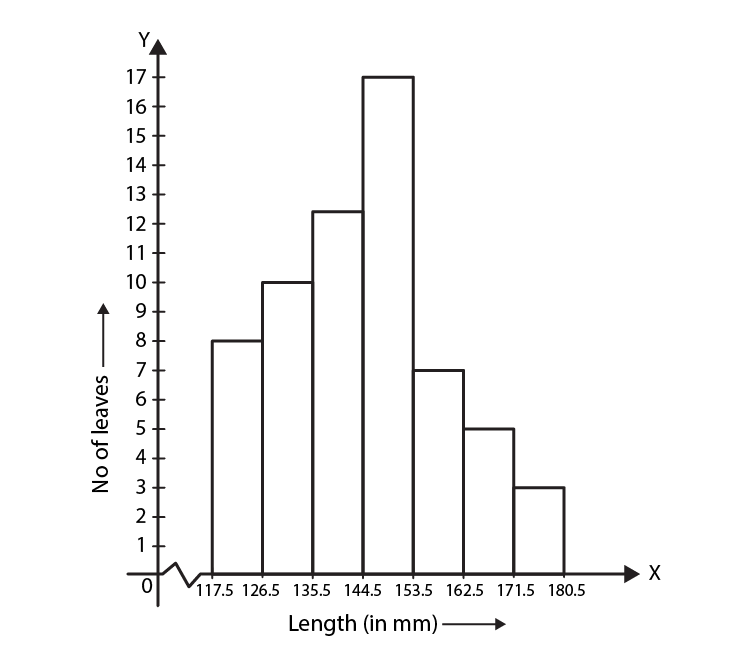The Exemplar Solutions can be used as a source tool for solving exercise questions of Chapter 14 present in the NCERT book and clear your doubts as well. Students can also refer to other study materials such as exemplar book, NCERT Solutions, notes, etc. to prepare for final exams. Solve some sample papers and previous year question papers to get an idea of the type of questions asked from statistics and probability and also marks held by it.

Download BYJU’S -The Learning App to get personalized videos explaining mathematics concepts such as Statistics and Probability and other related topics and experience a new method of learning.

## Frequently Asked Questions on NCERT Exemplar Solutions for Class 9 Maths Chapter 14

### Explain the concept of mean in Chapter 14 of NCERT Exemplar Solutions for Class 9 Maths?

The mean represents the average value of the dataset. It can be calculated as the sum of all the values in the dataset divided by the number of values. In general, it is considered as the arithmetic mean. Some other measures of mean used to find the central tendency are as follows:
Geometric Mean
Harmonic Mean
Weighted Mean
It is observed that if all the values in the dataset are the same, then all geometric, arithmetic and harmonic mean values are the same. If there is variability in the data, then the mean value differs
The solutions created by the faculty at BYJU’S help students to prepare for various competitive exams like JEE Main, JEE Advanced etc. including the annual exams. The PDF of solutions help students to understand the basic concepts and their applications in our daily lives.

### Where can I get the NCERT Exemplar Solutions for Class 9 Maths Chapter 14?

The chapter-wise NCERT Exemplar Solutions for Class 9 Maths Chapter 14 is available on BYJU’S. The solutions are designed by the teachers at BYJU’S after conducting vast research on each concept. PDFs of solutions are available which can be downloaded and used by the students to get their doubts cleared instantly. Each and every minute detail is given more importance in order to boost the exam preparation of Class 9 students.

### Write down the main topics of the NCERT Exemplar Solutions for Class 9 Maths Chapter 14.

The main topics of the NCERT Exemplar Solutions for Class 9 Maths Chapter 14 are –
1. Collection and presentation of data in graphical form
2. Measures of central tendency
3. The basic approach to probability and problems based on them